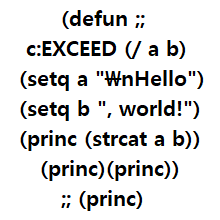# Text Position Z to layer## Recommended Poststhis routine is for polylines, is it possible to use this and
this time get TEXT position Z? instead on polyline elevations?

```   (defun c:LineElevation ( / c_doc c_lyrs ss clyr cnt pl_obj p_lst elev lyr_txt prms npoints)
c_lyrs (vla-get-layers c_doc)
clyr (getvar 'clayer)
);end_setq

(prompt "\nSelect Polylines : ")
(setq ss (ssget '((0 . "*POLYLINE"))))s
(cond (ss
(repeat (setq cnt (sslength ss))
(setq pl_obj (vlax-ename->vla-object (ssname ss (setq cnt (1- cnt)))))

(cond ( (vlax-property-available-p pl_obj 'elevation) (setq elev (vlax-get pl_obj 'elevation)))
(t
(setq p_lst (LD:sammlung_n (vlax-get pl_obj 'coordinates) 3)
);end_setq
)
);end_cond

(cond ( (< elev 650.0) (setq lyr_txt "NO_ELEV"))
( (= (rem (fix elev) 0.1) 0.1) (setq lyr_txt (strcat (itoa (0.1+(fix elev))))))
(t (setq lyr_txt (strcat (itoa (fix elev)))))
);end_cond

(if (not (tblsearch "layer" lyr_txt)) (vla-add c_lyrs lyr_txt))
(vlax-put-property pl_obj 'layer lyr_txt)
);end_repeat
)
);end_cond

(setvar 'clayer clyr)
(princ)
);end_defun

(defun LD:sammlung_n (o_lst grp / tmp n_lst)
(setq n_lst nil)
(cond ( (and o_lst (= (rem (length o_lst) grp) 0))
(while o_lst
(repeat grp (setq tmp (cons (car o_lst) tmp) o_lst (cdr o_lst)))
(setq n_lst (cons (reverse tmp) n_lst) tmp nil)
);end_while
)
);end_cond
(if n_lst (reverse n_lst))
);end_defun```

##### Share on other sitesThe vl 'coordinates does just that but is slightly different depending on the type of pline, A  LWpolyline will return x y and elev as a property, a 3dpoly will return x y z no elev. Splines something else again.

If I understand correct, as you use *polyline you could get 2d or 3d polys. So if you want co-ords of points make a new list for the 2d polys of the coordinates + elev. 3dpolys will be x y z.

##### Share on other sites

• 2 years later...BUMP my post up...
I needed this text position Z again. Im sorry

Is there a way to get the position Z of a TEXT (not MTEXT)

create a new layer and name it as position Z value

and move the text to that layer.

##### Share on other sites```(defun c:txtelev (/e el en l)
(setvar "osmode" 0)
(princ "\nSelect the text to obtain the elevation")
(while (not (setq sset (ssget ":S:E" '((0 . "TEXT")))))
(princ "\nSelect the text to obtain the elevation")
)
(vl-cmdf "_.layer" "_m" "position Z value" "_c" "5" "position Z value" "_l" "Continuous" "position Z value" "_s" "position Z value" "")
(setq e  (ssname sset 0)
en (entget e)
l  (assoc 8 en)
)
(princ (strcat "\nThe elevation is: " (rtos el 2 2)))
(entmod (subst (cons 8 "position Z value") l en))
(princ)
)```

##### Share on other sites```(vl-load-com)
(defun c:TextElevation (/ c_doc c_lyrs ss clyr cnt t_obj elev lyr_txt prms npoints)
c_lyrs (vla-get-layers c_doc)
clyr   (getvar 'clayer)
) ;end_setq

(prompt "\nSelect Texts : ")
(setq ss (ssget '((0 . "TEXT"))))

(cond
(ss
(repeat (setq cnt (sslength ss))
(setq t_obj (vlax-ename->vla-object (ssname ss (setq cnt (1- cnt)))))
(vlax-safearray->list
(vlax-variant-value (vlax-get-property t_obj 'InsertionPoint))
)
)
)
(cond
((< elev 650.0) (setq lyr_txt "NO_ELEV"))
((= (rem (fix elev) 0.1) 0.1)
(setq lyr_txt (strcat (itoa (0.1+ (fix elev)))))
)
(t (setq lyr_txt (strcat (itoa (fix elev)))))
) ;end_cond

(if (not (tblsearch "layer" lyr_txt)) (vla-add c_lyrs lyr_txt))
(vlax-put-property t_obj 'layer lyr_txt)
) ;end_repeat
)
) ;end_cond

(setvar 'clayer clyr)
(princ)
);end_defun```

you want this?

Edited by exceed
•1
##### Share on other sites23 minutes ago, exceed said:
```(defun c:TextElevation (/ c_doc c_lyrs ss clyr cnt t_obj elev lyr_txt prms npoints)
c_lyrs (vla-get-layers c_doc)
clyr   (getvar 'clayer)
) ;end_setq

(prompt "\nSelect Texts : ")
(setq ss (ssget '((0 . "TEXT"))))

(cond
(ss
(repeat (setq cnt (sslength ss))
(setq t_obj (vlax-ename->vla-object (ssname ss (setq cnt (1- cnt)))))
(vlax-safearray->list
(vlax-variant-value (vlax-get-property t_obj 'InsertionPoint))
)
)
)
(cond
((< elev 650.0) (setq lyr_txt "NO_ELEV"))
((= (rem (fix elev) 0.1) 0.1)
(setq lyr_txt (strcat (itoa (0.1+ (fix elev)))))
)
(t (setq lyr_txt (strcat (itoa (fix elev)))))
) ;end_cond

(if (not (tblsearch "layer" lyr_txt)) (vla-add c_lyrs lyr_txt))
(vlax-put-property t_obj 'layer lyr_txt)
) ;end_repeat
)
) ;end_cond

(setvar 'clayer clyr)
(princ)
);end_defun```

you want this?

thank you so much for this sir! just what I needed

## Join the conversation

You can post now and register later. If you have an account, sign in now to post with your account.
Note: Your post will require moderator approval before it will be visible.Unfortunately, your content contains terms that we do not allow. Please edit your content to remove the highlighted words below.

×   Pasted as rich text.   Restore formatting

Only 75 emoji are allowed.